# C++ Program to Compute Sum of n^2/(n-1) Series

In mathematics, there are many series whose sum is calculated using computer programs to reduces the effort of doing enormous computations. This example program computes the sum of series (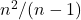). The user has to input the value of n.

To write such programs you must be careful about two things – accuracy and type conversions. Accuracy means correct to at least 3 decimal places because series involves real values. A small difference can produce wrong results.

Sometimes we need to do arithmetic between an integer value and a float value. In such cases, to get the correct value of the expression to convert both operands into the same type using C++ type cast operator.

Before you begin, learn basics of C++ programming. Continue reading if you are familiar with the basics.

## Problem Definition

We want to write a program that computes the sum of the following series and prints an output to the console.

n^2/(n-1)

When the program runs, the user provides the value of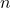and the program computes the sum of all values up to n. The program cannot have a value less than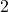because any value less thanis undefined.

For example,

\begin{aligned}
&if \hspace{5px} n = 1\\\\
&= 1^2/(1 - 1)\\\\
&= 1/0\\\\
&= Undefined
\end{aligned}

To understand the problem let us do a manual computation and verify the results using our example program. Suppose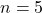, then

\begin{aligned}
&= \frac{2^2}{(2 - 1)} + \frac{3^2}{(3-1)} + \frac{4^2}{(4-1)}+\frac{ 5^2}{(5-1)}\\\\
&= 4/1 + 9/2 + 16/3 + 25/4\\\\
&= 20.08
\end{aligned}

You can use a calculator or do fraction addition. The final sum of the series is given below.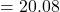Now, you need to verify the output by running the C++ program for the sum of the series given below.

## Program Source Code

#include <cstdlib>
#include <iostream>

using namespace std;
int main(){

int n,i;
double sum;

//initialize sum to 0
sum = 0.0;

cout << "Enter the value of n:";
cin >> n;

//Compute the value of the sum, but the equation has a restriction.
//The value of n should not be less than 2
for(i=2;i<=n;i++)
{
sum = sum + float(i * i)/float(i-1);
}

//Printing the Output to Console
cout << "\n";
cout << "Sum of the Series n^2/(n-1) =" << " " << sum << endl;
cout << "\n";

system("PAUSE");
return EXIT_SUCCESS;
}

## Output

The output of the program is the same what we received using manual computation method. Therefore, the program works correctly. You may try different inputs.

Enter the value of n:5
Sum of the Series n^2/(n-1) = 20.0833
Press any key to continue . . .